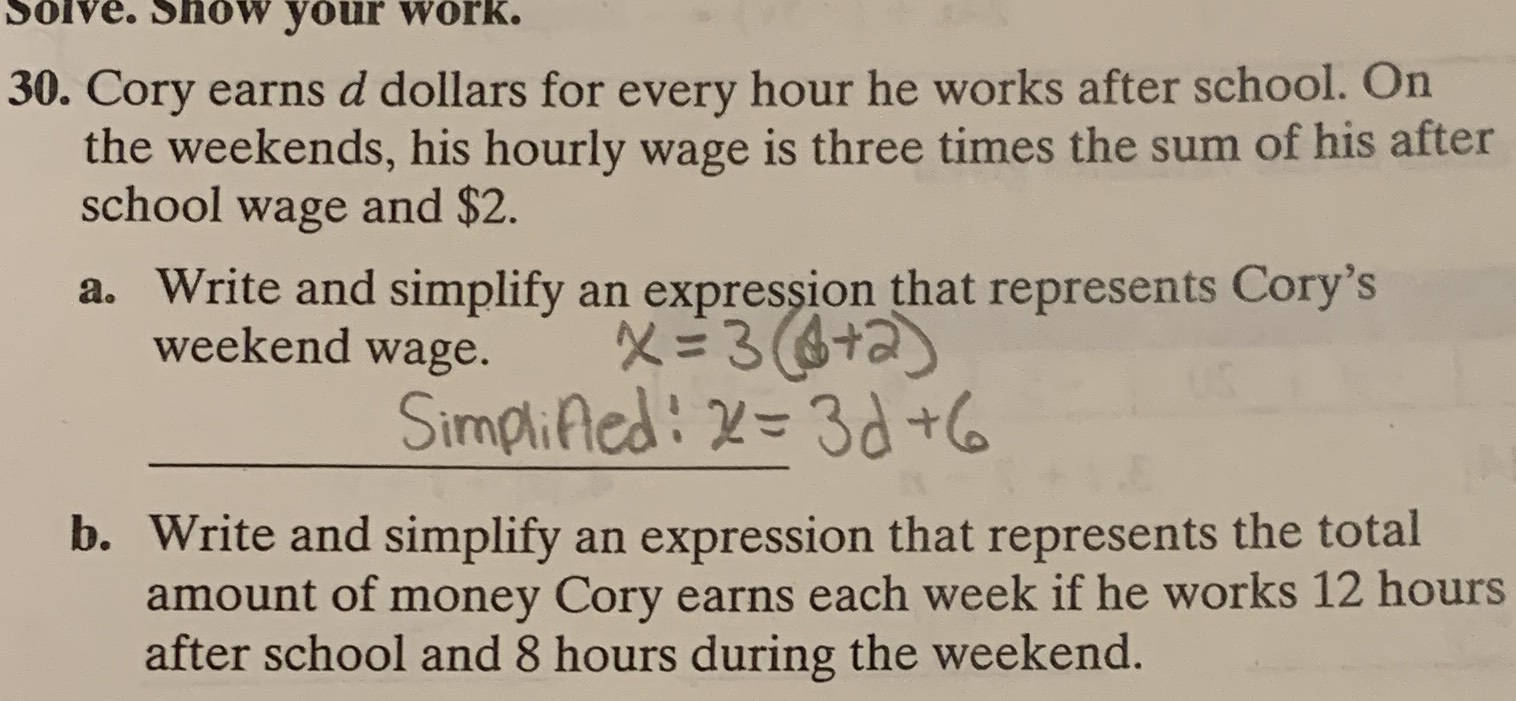### ¿Todavía tienes preguntas de matemáticas?

Pregunte a nuestros tutores expertos
Algebra
Pregunta30. Cory earns $$d$$ dollars for every hour he works after school. On the weekends, his hourly wage is three times the sum of his after school wage and $$\ 2 .$$

a. Write and simplify an expression that represents Cory's weekend wage. $$x = 3 ( \phi + 2 )$$

Simplificd: $$x = 3 d + 6$$

b. Write and simplify an expression that represents the total amount of money Cory earns each week if he works $$12$$ hours after school and $$8$$ hours during the weekend.

a) Cory's weekend wage is x=3(d+2)

Simplifying will give x=3d+6

b) Total amount of money y=12d+8(3(d+2))

y=12d+24d+48

y=36d+48

Solución
View full explanation on CameraMath App.